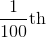# SSAT Elementary Level Verbal : Measurement

## Example Questions

2 Next →

### Example Question #521 : Analogies

Complete this analogy.

Foot is to inch as __________.

goose is to geese

red is to blue

gallon is to cup

centimeter is to meter

foot is to feet

gallon is to cup

Explanation:

The analogy compares first a relatively large measurement to a relatively small measurement. There are two answer options that meet this criteria. "Centimeter is to meter" is an enticing choice that compares a relatively small measurement to a relatively large measurement; therefore, the relationship is reversed, and it's not quite right. "Gallon is to cup," although not a measurement of length like "foot is to inch" and "centimeter is to meter," correctly compares a relatively large measurement to a relatively small measurement, so it is the correct answer.

### Example Question #522 : Analogies

Complete this analogy.

Inch is to foot as second is to __________

mile

day

minute

pound

gallon

minute

Explanation:

There are twelve inches in a foot and three feet in a yard, but you don't need to know that specifically to answer this question. An inch is a unit of measurement that makes up a foot, so to solve this analogy, you have to determine which of these answer choices is a unit that is made up of seconds. There are sixty seconds in a minute, so "minute" is the correct answer.

### Example Question #523 : Analogies

Analogies: Complete this analogy.

Kilogram is to weight as centimeter is to __________.

mass

length

distance

time

speed

length

Explanation:

A "kilogram" is a unit of measurement used to measure how much something weighs, so to solve this analogy you have to determine what a "centimeter" is used to measure. The correct answer is that a "centimeter" is a unit of measurement that measures length, it isof a meter.

### Example Question #524 : Analogies

Complete this analogy.

Celsius is to Fahrenheit as __________ is to kilograms.

pounds

meters

inches

kelvin

yards

pounds

Explanation:

"Celsius" and "Fahrenheit" are both units for measuring temperature. A "kilogram" is a unit for measuring weight. So, which of these answer choices is also a unit for measuring weight? The correct answer is "pounds." Additionally, "meters," "yards," and "inches" are all units for measuring length; "kelvin" is another unit for measuring temperature.

### Example Question #521 : Analogies

Complete this analogy.

Mile is to kilometer as pound is to __________.

weight

length

gallon

liter

kilogram

kilogram

Explanation:

"Mile" and "kilometer" are both units of "length," they are used to measure how long or how far something is. A "pound" is used to measure "weight," or how heavy something is. So, which of these answer choices is also a unit of measurement used to measure "weight?" The correct answer is "kilogram." To provide additional help, "liter" and "gallon" are both used to measure how much "volume" a liquid has, or how much liquid there is in a container.

### Example Question #526 : Analogies

Complete this analogy.

Inch is to __________ as gram is to weight.

meter

time

length

foot

size